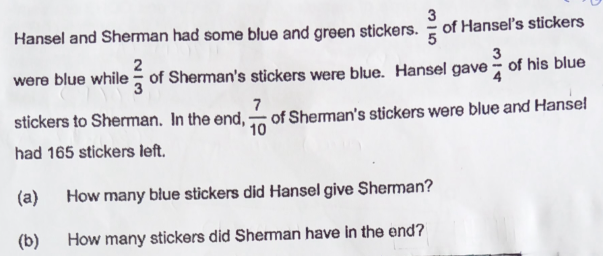# QuestionThank you!

H    [B][B][B][G][G]   <—–(3/4) x (3/5) = 9/20  => 9u blue stickers given away, total parts = 20u

<-12u–><-8u->

S     [       B         ][       B         ][       G        ]

(a)  From H, stickers left = 20u-9u =11u.

11u = 165 => u = 15

Blue stickers that H has given away = 9u = 9 x 15 = 135

(b)  S’s stickers      Blue               Green

Before               2s                      s

From H           +135

After                  7p                    3p

s = 3p

2(3p) + 135 = 7p

p = 135

Hence Sherman has 10p = 1350  stickers in the end.